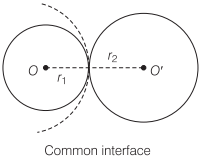# Surface Energy | Definition, Formula, Units – Surface Tension

Surface Energy Definition:
If we increase the free surface area of a liquid, then work has to be done against the force of surface tension. This work done is stored in liquid surface as potential energy.

We are giving a detailed and clear sheet on all Physics Notes that are very useful to understand the Basic Physics Concepts.

## Surface Energy | Definition, Formula, Units – Surface Tension

This additional potential energy per unit area of free surface of liquid is called surface energy.

Surface Energy Formula:

Surface Energy = $$\frac{\text { Energy }}{\text { Area }}$$

Surface Energy (E) = S x ΔA
where, S = surface tension and ΔA = increase in surface area.

Unit of Surface Energy:
SI Unit of Surface Energy is N/m

Surface Energy Dimensional Formula:
Dimensional Formula is M1L2T-2

(i) Work Done in Blowing a Liquid Drop:
If a liquid drop is blown up from a radius r1 to r2, then work done for that is

W = S . 4π(r2² – r1²)

(ii) Work Done in Blowing a Soap Bubble:
As a soap bubble has two free surfaces, hence work done in blowing a soap bubble so as to increase its radius from r1 to r2 is given by

W = S . 8π(r2² – r1²)

(iii) Work Done in Splitting a Bigger Drop into n Smaller Droplets:
If a liquid drop of radius R is split up into n smaller droplets, all of same size, then radius of each droplet

r = R . (n)-1/3
Work done, W = 4πS(nr² – R²) = 4πSR² (n1/3 – 1)

(iv) coalescence of Liquid Drops:
If n small liquid drops of radius r each combine together so as to form a single bigger drop of radius R = n1/3 . r, then in the process energy is released. Release of energy is given by

ΔU = S . 4π(nr² – R²) = 4πSr²n (1 – n-1/3)

Excess Pressure due to Surface Tension
(i) Excess pressure inside a liquid drop = $$\frac{2 S}{R}$$
(ii) Excess pressure inside an air bubble in a liquid = $$\frac{2 S}{R}$$
(iii) Excess pressure inside a soap bubble = $$\frac{4 S}{R}$$
where, S = surface tension and R = radius of drop/bubble.

Excess Pressure in Different Cases1. Work done in spraying a liquid drop of radius R into n droplets of radius r = S x Increase in surface area

= 4πSR3$$\left(\frac{1}{r}-\frac{1}{R}\right)$$

Fall in temperature,

Δθ = $$\frac{3 S}{J}\left(\frac{1}{r}-\frac{1}{R}\right)$$

where, J = 4.2 J/cal.

2. When n small drops are combined into a bigger drop, then work done is given by

W = 4πR²S (n1/3 – 1)

Temperature increase,

Δθ = $$\frac{3 S}{J}\left(\frac{1}{r}-\frac{1}{R}\right)$$

3. When two bubbles of radii r1, and r2 coalesce into a bubble of radius r isothermally, then

r² = r1² + r2²

4. When two soap bubbles of radii r1 and r2 are in contact with each other, then radius r of common interface$$\frac{1}{r}=\frac{1}{r_{1}}-\frac{1}{r_{2}}$$ or r = $$\frac{r_{1} r_{2}}{r_{2}-r_{1}}$$

### Formation of a Single Bubble

1. If two bubbles of radius r1 and r2 coalesce isothermally to form a single bubble of radius r under external pressure p0, then surface tension of the liquid

S = $$\frac{p_{0}\left[r^{3}-r_{1}^{3}-r_{2}^{3}\right]}{4\left[r_{1}^{2}+r_{2}^{2}-r^{2}\right]}$$

2. Pressure inside bubbles are

p1 = $$\left(p_{0}+\frac{4 S}{r_{1}}\right)$$, p2 = $$\left(p_{0}+\frac{4 S}{r_{2}}\right)$$, p3 = $$\left(p_{0}+\frac{4 S}{r}\right)$$

Also, p1V1 + p2V2 = p3V3

where,
p1, V1 are pressure and volume of first bubble,
p2, V2 are pressure and volume of second bubble and
p3, V3 are pressure and volume of new bubble.

Surface Tension:
In Physics, the tension of the surface film of a liquid because of the attraction of the surface particles by the bulk of the liquid, which tries to minimize surface area is called surface tension. When the surface of the liquid is strong enough, then surface tension is applicable. It is strong enough to hold weight.